## Example Questions

← Previous 1

### Example Question #1 : Other Exponents

What is the sum of the solutions of the equation x2-2x-8=0?

-2

-6

-10

6

2

2

Explanation:

The equation factors to (x-4)(x+2)=0, indicating that the roots are x=-2,4. The sum of these two roots is 2.

### Example Question #2 : How To Use Scientific Notation

What is the number 350,000,000 in scientific notation?

3.5 x 108

3.5 x 109

3.5 x 106

3.5 x 107

3.5 x 105

3.5 x 108

Explanation:

350,000,000 = 3.5 x 108 (exponent is equal to the number of decimal points shifted)

### Example Question #1 : Other Exponents

Given the equation 2xy + 4x² - y = 2x, if x = 2, what does y equal?

-4

4

2

0

-4

Explanation:

We plug in the value given for x and solve for y.

2xy + 4x² - y = 2x

2(2)y + 4(2)² - y = 2(2)

4y + 4(4) - y = 4

4y - y + 16 = 4

3y = -12

y = -4

### Example Question #1 : How To Use Scientific Notation

How do you express 657,800 in scientific notation?

65.78 × 104

65.78 × 106

6.578 × 104

65.78 × 103

6.578 × 103

65.78 × 104

Explanation:

657800 = 6.578 × 105

OR

657800 = 65.78 × 104

Exponent on 10 is the number of decimal points over the number has shifted.

### Example Question #2 : How To Use Scientific Notation

2 × 100 + 3 × 102 + 4 × 104 = ?

40,320

4,032

400,032

432

40,302

40,302

Explanation:

2 × 100 + 3 × 102 + 4 × 104 =

2 + 300 + 40,000 = 40,302

### Example Question #1 : How To Use Scientific Notation

Give this number in scientific notation: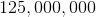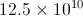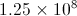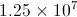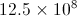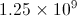Explanation:

There are six zeros and two more number places, for a total of eight, so 125,000,000 is equivalent to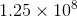.

### Example Question #2 : Other Exponents

Simplify the following expression: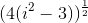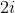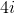Explanation: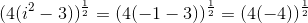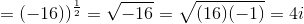### Example Question #1 : How To Use Scientific Notation

What is the number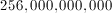in scientific notation?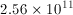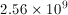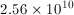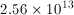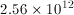Explanation: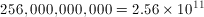The exponent is equal to the number of decimal points shifted.

### Example Question #1 : How To Use Scientific Notation

Convert from scientific to decimal notation: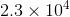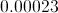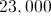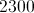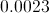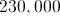Explanation:

In this case the exponent is positive hence you have to move the decimal point 4 places to the right from where it is in the problem giving us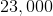### Example Question #1 : How To Use Scientific Notation

Convert from decimal to scientific notation: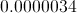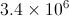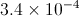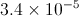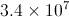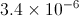Explanation:

In this case you have to move the decimal point 6 places to the right. When you move the decimal point to the right the exponent becomes negative.  Similarly when you move the decimal point to the left the exponent is positive.  Also, to the left of the decimal place there can be only one digit.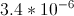← Previous 1

### All ACT Math Resources